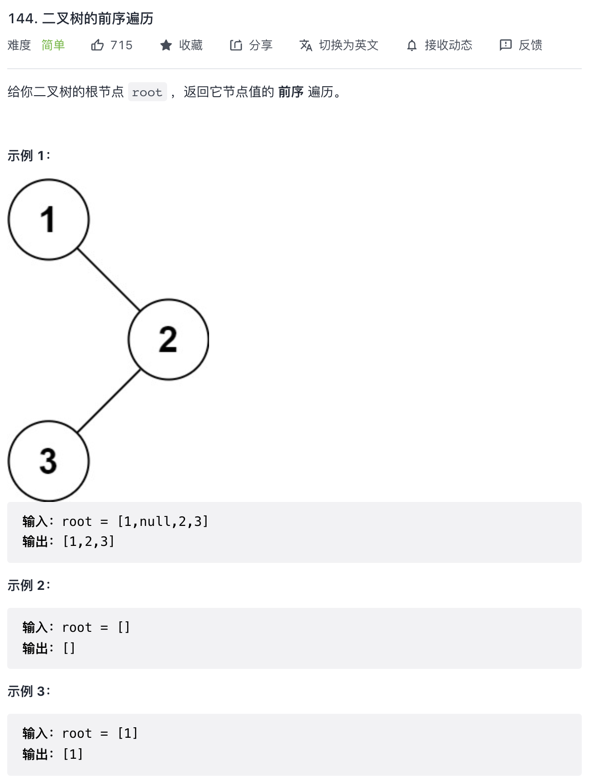leetcode：二叉树的前序遍历

### 描述：### 题解：#### 思路一：使用递归

``````function traversal(root)
``````

``````function traversal(root){
if (root == null) {
return
}
}
``````

``````function preorderTraversal(root) {
const res = []
traversal(root)
return res
function traversal(root) {
if (root == null) {
return
}
res.push(root.val)
traversal(root.left)
traversal(root.right)
}
}

``````

#### 思路二：使用栈``````function preorderTraversal(root) {
if (root == null) {
return []
}
const res = []
//模拟一个栈
const stack = []
stack.push(root)

while (stack.length) {
while (root) {
//进栈的同时保存遍历的结果
stack.push(root)
res.push(root.val)
root = root.left
}
//如果没有左节点了，出栈，处理右节点
root = stack.pop()
root = root.right
}
return res
}

``````